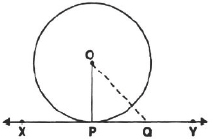# CBSE Class 10 Math, Circles

Class X Math
Notes for Facts that Matter
Facts that Matter
Tangent to a Circle
A tangent to a circle is a line that touches the circle at only one point.
Theorem 1
The tangent at any point of a circle is perpendicular to the radius, through the point of contact.
Proof: We have the centre O of the given circle and XY is the tangent to the circle at a point P.
Let us take a point Q on Xy other than P, Join OQ.Obviously, Q lies outside the circle.
i.e., OQ > OP
Since, all the points on XY, except P lies outside the circle.
i.e., OP is smaller than all the distance of the point O from XY.
i.e., OP is the smallest distance of O from XY.
i.e., OP ⊥ XY